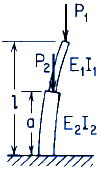Related Resources: calculators

Stepped straight bar under end load P and intermediate load P2; upper end free, lower end fixed.NOTATION:

P1 = Load (force) (lbs, N);
p' = critical unit load (force per unit length), (lbs, N);
T' = critical torque (force-length A.R.) (in-lbs, N-mm);
M' critical bending moment (force-length);
E = modulus of elasticity (force per unit area) (psi, Pa);
I = moment of inertia of cross section about central axis perpendicular to plane of buckling ( in4, mm4 )
l = Length of bar (in, mm),
K1 = Derived from ratios as follows (see table):
1.) ( E2 I2 ) / ( E1 I1 )
2.) a / l
3.) P2 / P1

Formulae:

P'1 = ( K1 π2 E1I1 ) / l2

Where:

References:

Roark's Formulas for Stress and Strain